## Agricultural Science Digest

• Chief EditorArvind kumar

• Print ISSN 0253-150X

• Online ISSN 0976-0547

• NAAS Rating 4.75

• SJR 0.156

Frequency :
Bi-monthly (February, April, June, August, October and December)
Indexing Services :
BIOSIS Preview, Biological Abstracts, Elsevier (Scopus and Embase), AGRICOLA, Google Scholar, CrossRef, CAB Abstracting Journals, Chemical Abstracts, Indian Science Abstracts, EBSCO Indexing Services, Index Copernicus
Agricultural Science Digest, volume 43 issue 2 (april 2023) : 170-175

# Predictive Modelling of Height and Diameter Relationships of Himalayan Chir Pine

Afshan Tabassum1,*, M. Iqbal Jeelani1, Manish Sharma1, Khalid Ul Islam Rather1, Imran Rashid1, Mansha Gul1
1Division of Statistics and Computer Science, Sher-e-Kashmir University of Agricultural Sciences and Technology of Jammu, Chatha-180 009, Jammu and Kashmir, India.
• Submitted17-01-2022|

• Accepted11-07-2022|

• First Online 23-07-2022|

Cite article:- Tabassum Afshan, Jeelani Iqbal M., Sharma Manish, Rather Islam Ul Khalid, Rashid Imran, Gul Mansha (2023). Predictive Modelling of Height and Diameter Relationships of Himalayan Chir Pine . Agricultural Science Digest. 43(2): 170-175. doi: 10.18805/ag.D-5555.
Background: In this study 11 height and diameter prediction models were fitted and evaluated for Himalayan Chir pine (Pinus roxburghii) in Jammu region of UT J and K (India). The data were collected from 50 permanent sample plots in uneven aged stands of Pinus roxburghii and total of 500 individual tree height and diameter measurement were used for this study. At initial stage all the models fitted resulted in significant coefficients, besides various selection criteria’s were also used to test the predictive performance of fitted models. The results of these criteria were generated from various libraries of R studio (version 3.5.1, 2018). The models were further cross validated and results revealed Manfred (MG) and Michaelis-Menten2 (MJ) models described the highest amount of height variation in terms of fit statistics and more crucially with lowest prediction error rate as compared to other models.
Methods: The study was carried out in Jammu region of UT J and K (India). Data used in this study were collected on 50 permanent sample plots of 0.25 ha in size.In order to achieve stipulated objectives, Height diameter data on 500 trees from Jammu forest division was utilized in this study.
Result: The summary statistics of height and diameter variable and the overall summary of the coefficients of various height and diameter models in Jammu forest division are presented. Almost all the coefficients of the statistical models were statistically significant which is an indication that fitted models are capturing the height diameter relationship an important aspect in context to biological realism.
Pinus roxburghii (Sarg.) is named after William Roxburgh, also known as long leaf pine or chir pine and belongs to Pinus section diploxylon, characterized by hard timber and two xylem bundles, In India it occurs naturally in the outer ranges and valleys of the Himalayas; on the ridges of Shiwalik hills flanking the Himalayas, from altitude of 500-2500 m and covers approximately 6,77,813 ha area in the states viz. Himachal Pradesh, Jammu and Kashmir and Uttarakhand. Economically, pines are important source of wood, paper, resins, charcoal, food (particularly seeds) and ornamentals (dried cones etc.). In India major share of resin production comes from Himachal Pradesh, Uttarakhand and Jammu and Kashmir (Sharma, 2001). Chir pine provides a variety of wide ranged goods and services to the people both at local and national level. Hence its management is very important which requires the knowledge of height and diameter relationships. Various studies have revealed that these models adequately fit height diameter relationships, but sometimes they may involve various types of errors, while dealing with varying aged stands. In order to correct these situations the techniques of validation are adopted which is used when one wants to estimates how accurately statistical models will perform in practice. The idea of validation was originated in 1930s (Larson, 1931) and further generalised by (Mosteller and Turkey, 1968). In this study fitting and validation of models were carried out in R studio, which was developed in the year (2011) by J.J Allaire (R Development Core Team 2016). R has become the de-facto system for statistical analysis and graphics developed by Ross Ihaka and Robert Gentleman in the year 1995 andit handles large data sets much better than it used to, say even a decade earlier and has become a lot more versatile. Jeelani et al., (2015) compared linear regression model based on various sampling techniques like simple random sampling (SRS), systematic sampling (SYS) and rank set sampling (RSS) under validation technique (LOOCV). The result revealed that there was consistency in the measure of R2, Adj R2 and RMSE in case of RSS as compared to SRS and SYS. Results were also supported with an empirical study involving a real data set generated from Pinus wallichiana taken from Kashmir, India. Hasanzad et al., (2016) studied the relationship between height and diameter of velvet maple. A complete list of the selected height diameter models was used and nineteen candidate models were considered. Various criteria were chosen and applied to evaluate the predictive performance of the models. Jeelani et al., (2017) studied k-fold cross validation method for performance evaluation of different regression models. Regression analysis was used to study the relationship between fodder yield (dependent variable) and other parameters. The models were validated for its adequacy through different criteria. Jeelani et al., (2018), Kumar et al., (2021), Harsh et al., (2022) studied the relationship between fodder yield and other parameters of Grewia optiva in Jammu region. On the basis of set criteria, the models were ranked. This study aim at fitting, evaluating and validating different statistical models so that they can be used to predict height and diameter relationship in pinus roxburghii of Jammu region of UT J and K (India).
The study was carried out in Jammu region of UT J and K (India). Data used in this study were collected on 50 permanent sample plots of 0.25 ha in size.In order to achieve stipulated objectives, Height diameter data on 500 trees from Jammu forest division was utilized in this study. The forest composition of Chir in terms of area in Jammu forest division is given in Table 1.

In view of the above various height and diameter statistical models were used to study the tree height and diameter relationships of chir trees in each division. The functional form of the models used in this study is as follows: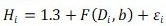Where,
Hi = ith observation of the response variable tree height (m).
Di = ith observation of the predictor variable diameter at breast height (cm).
b = A vector of model parameters.
ei = Unexplained error, that it is assumed to be independent and normally distributed with mean zero and a constant variance.

A constant, 1.3 (which is bh in models below) is added to the model to avoid prediction of a Hi less than 1.3 m when Di approaches zero.
Based on the above functional form eleven height diameter models were used in this study, whose description is given in Table 2.

Then adequacy of the fitted models was tested by using different selection criteria like AIC, BIC, RMSE, (Adj. R2), etc. which is given below: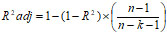Where,
R2 = Coefficient of determination.
n = Number ofobservation and k is number of parameters.

Root mean square error (RMSE)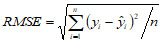Where,
n = Total number of observations.
yi = Actual observation.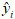= Predicted value.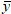= Mean of observed value.

Mean absolute error (MAE)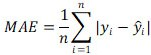Where,
n = Total number of observations.
yi = Actual observation.= Predicted value= Mean of observed value, Raghav et al., (2022).

Akaike information criterion (AIC)

AIC = n × In(RMSE) + 2k

Where,
n = Number of observations.
k = Number of parameters.

Bayesian information criterion (BIC)

BIC = -2In(l) + In(n) × k

Where,
l = Maximum likelihood value of the model.
k = Number of degrees of freedom (or independently adjusted parameters) in the model.
n = Number of observations (total sample size).

Residual standard error (RSE)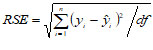Where
y = The observed value.= The predicted value.
df = The degrees of freedom.

Further, the assumption of normality of errors of fitted models was accessed by Shapiro Wilk test of significance. Then to check the predictive performance of the fitted models cross validation method was used and data was randomly partitioned into training and testing sets, where 80 per cent f data was used for training and the remaining 20 per cent of the data for testing. Statistical analysis of above models was carried out in R studio (version 3.5.1, 2018) utilising its various libraries. Further the function geom_line and ggtitle of R studio was used for creating these GG-plots.
The summary statistics of height and diameter variable and the overall summary of the coefficients of various height and diameter models in Jammu forest division are presented in Table 3 and 4. A glance of Table 4 disclose that almost all the coefficients of the statistical models were statistically significant which is an indication that fitted models are capturing the height diameter relationship an important aspect in context to biological realism. Also this table revealed the performance of height diameter  models across the forest division utilizing various selection criteria like RMSE, MAE, BIAS, RSE, AIC, BIC, MAE, RMSE, R2 and Adj. R2. Among significant fitted models MG (Manfred N3) height diameter model was found out to be the best model with lower values of RMSE, MAE, AIC and BIC, also with non-significant Shapiro Wilk test for normality of errors.Table 4: Estimates of parameter and performance criteria of fitted height and diameter models in Jammu forest division.

In order to access the predictive performance of fitted models, cross validation method (80:20) was adopted, utilizing 80 per cent data as training set and remaining 20 per cent as testing set. Table 5 reveal the performance criteria of models under training and testing data set in case of Jammu forest division. A perusal of Table 5 revealed that MG (Manfred N3)  and MJ (Michaelis-Menten2) performed better across both data sets in Jammu forest division as both the models resulted in lower values prediction error rates (PER).Table 5: Evaluation of height diameter models under training and testing data sets in Jammu forest division.

Apart from this all the models were ranked based on prediction error rates (PER), a perusal of which is given in Table 6.

The evaluation of height diameter models with respect to training and testing data sets were also visualized graphically with the help of library (ggplot2) in R studio. A perusal of GGplots across Jammu forest division with respect to various performance criteria are given in Fig 1, 2 and 3.

At primary stage data on height and diameter variables of 500 Chir Pine trees were takenand accordingly eleven height diameter models were fitted on the data. Initial analysis revealed that almost all the parameters across the models were found out to be significant. At second stage various selection criteria’s like RMSE, MAE and BIAS etc. were used to study the predictive performance of fitted height diameter models. Various functions of R were utilized with the help of various libraries like minpack.lm, Metrics, caret, tidyverse and nlme of R to generate the results of various selection criteria’s. It was revealed that RSE ranged from 4.23 to 6.74, while as AIC and BIC ranged from 438.34 to 670.28 and 443.55 to 678.09. Further MAE and RMSE ranged from 2.48 to 4.78 and 4.19 to 6.70, while as Adj.R2 and R2 from 0.66 to 0.85 and 0.66 to 0.88. Based on these results MG (Manfered N3) and MJ (Michaelis-Menten2) height diameter models performed well in Jammu forest division among all the models used in this study. Further the prediction error rate (PER) ranged from 0.29 to 0.77 and 0.25 to 0.65 in case of training and testing data sets, which revealed that results were in favour of MG (Manfered N3) and MJ (Michaelis-Menten2) model as both models resulted in lower values of PER, also have lowest values of RMSE, MAE, AIC, BIC and higher values of Adj.R2. Further the function geom_line and ggtitle of R studio was used for creating these GG-plots. Predicted height on Jammu forest data sets based on the fitted models were also plotted using library (ggplot2).
The relationship between tree height and diameter is an important element of forest structure that can used to describe the strategies of tree species among individuals at a particular point, to estimate timber volume and site index and is also an important variable in growth and yield modelling. Hence development of the simple and most accurate height and diameter models enables forest managers to assemble the reliable prediction of tree height in forests. To achieve this, eleven height diameter models were used in this study. Results revealed that theselection of best and most accurate models should be based on the predication error rate (PER) which was used in this study. Among the eleven models, MG (Manfred N3) and MJ (Michaelis-Menten2) recognized as the best fit and accounted for the highest amount of total height variations and more crucially with lowest prediction error rate and both models appeared to be biologically more realistic. Hence it is proposed that height diameter relationship should be known in advance for any forest policy and planning as the information about such relationships is essential for better understanding of tree stand dynamics.
None.

View all (0)